### Special Relativity with Geometric Algebra - Spacetime Algebra

#### Paths of objects

There are different ways to understand and formalize the paths objects take and how they move over time.
##### Position over time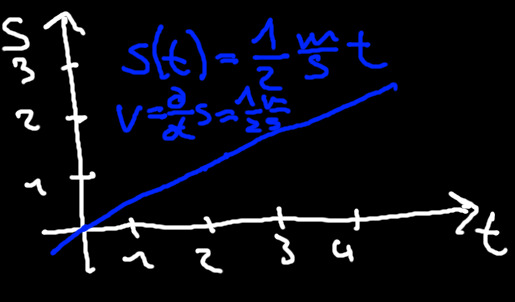Figure 1 - Path of an object with constant velocity. X-axis: time. Y-axis: space.
In physics we often draw the path an object takes over time in a diagram where time is on the x-axis and space is on the y-axis. Figure 1 shows such a diagram. For an object with a constant velocity of one half meters per second we have the following equations
$\begin{array}{}\text{(1)}& \begin{array}{rl}s\left(t\right)& =\frac{1}{2}\frac{\mathrm{m}}{\mathrm{s}}t\\ v\left(t\right)& =\frac{\partial }{\partial t}s\left(t\right)=\frac{1}{2}\frac{\mathrm{m}}{\mathrm{s}}\end{array}\end{array}$
##### Position over time - flipped space and time axes
In relativity, the space and time axes are usually flipped, so we space is on the x-axis and time is on the y-axis. We will also follow this standard practice. Doing this our diagram will look like thisFigure 2 - Path of an object with constant velocity, X-axis: space. Y-axis: time.
Since we are using geometric algebra, we will use vectors to formulate paths of objects instead. We will have the usual spatial basis vectors${e}_{x},{e}_{y},{e}_{z}$, but why not introduce a basis vector${e}_{t}$for time too? After all, in our diagram these don't really look any different. If we do this we have four basis vectors in total and instead of just space we now have spacetime.
##### Parameterized paths with vectors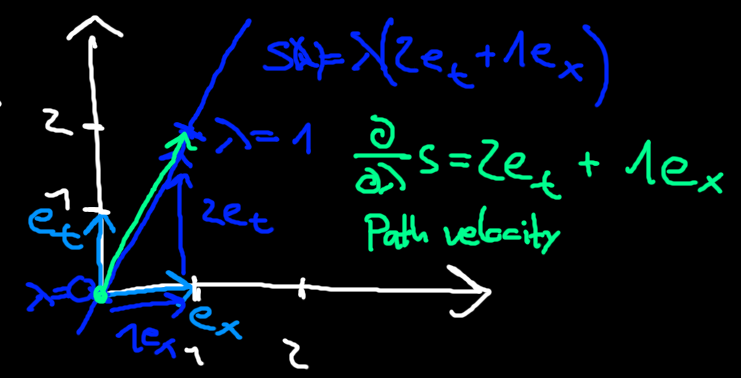Figure 3 - Light-blue: Orthonormal basis vectors for time and space. Blue: Vector path of an object parameterized by$\lambda$. Green: Path velocity of the blue path.
For our previous example, for every step in the space direction we take two steps in the time direction. So an unnormalized direction vector for the path is given by$2{e}_{t}+1{e}_{x}$. We can now introduce a parameter$\lambda$that sweeps out our path
$\begin{array}{}\text{(2)}& s\left(\lambda \right)=\lambda \left(2{e}_{t}+1{e}_{x}\right)\phantom{\rule{0ex}{0ex}}\end{array}$
We can also calculate a path velocity by taking the derivative with respect to our path parameter$\lambda$
$\begin{array}{}\text{(3)}& v=\frac{\partial }{\partial \lambda }s\left(\lambda \right)=2{e}_{t}+1{e}_{x}\end{array}$
The path velocity is always tangent to the path.
Note that the parameterization for our path is somewhat arbitrary. We could just as well have multiplied by path by a constant and have gotten the same path. What happens to the path velocity when we multiply our path by a constant factor$k$?
$\begin{array}{}\text{(4)}& \begin{array}{rl}{s}_{k}\left(\lambda \right)& =\lambda k\left(2{e}_{t}+1{e}_{x}\right)\\ {v}_{k}& =\frac{\partial }{\partial \lambda }{s}_{k}\left(\lambda \right)=k\left(2{e}_{t}+1{e}_{x}\right)\end{array}\end{array}$
The path velocity also receives the constant factor$k$. In order to fix this arbitrary path parameter, we choose the length of the path velocity to be the speed of light, ie.$||v|{|}^{2}={v}^{2}={c}^{2}$. The path parameter which we generally called$\lambda$is then called the proper time$\tau$.
Our path parameterized by proper time is then$s\left(\tau \right)$and we introduce a short-hand notation for the derivative with respect to proper time$\stackrel{˙}{s}\left(\tau \right)=\frac{\partial }{\partial \tau }s\left(\tau \right)$. Because of our definition for proper time we now have${\stackrel{˙}{s}}^{2}={c}^{2}$. In many places the same equation but with$1$on the right-hand side is seen. This is because often the choice$c=1$is made.

#### Spacetime events

Another thing we want to look at is what the points in our spacetime, such as the points on our paths, represent. A point contains a time coordinate and three space coordinates. Points in spacetime are also called events because of this.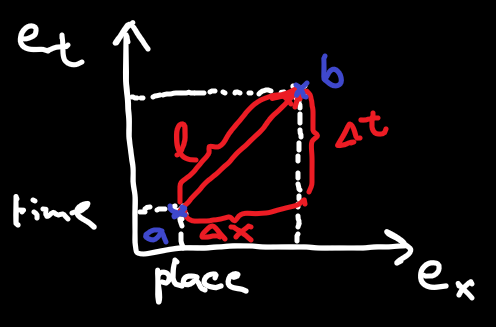Figure 4 - Time as another dimension and spacetime events
An event$a$as shown in the diagram could be "I left home at 8am" with the position being home and the time being 8am. Another event$b$could then be "I arrived at work at 9am" with position work and time 9am. We can now form difference vectors again. For this example assume home and work are 10km apart in the x direction. Then we have a difference vector
$v=3600\mathrm{s}{e}_{t}+10\mathrm{km}{e}_{x}$
Does this expression make sense? The first problem we can notice is that the units don't match up. How do we add kilometers (spatial distance) and seconds (time difference)? To remedy this, we could multiply the time component by a constant speed as that would result in a distance. Why not choose the speed of light$c$? We now the following expression with the correct units
$v=c3600\mathrm{s}{e}_{t}+10\mathrm{km}{e}_{x}$
Well we got around the unit issue, although we did not justify the multiplication by$c$very well yet. The true justification for it will come soon.

#### More spacetime paths

Let's take a look at some more types of paths in spacetime.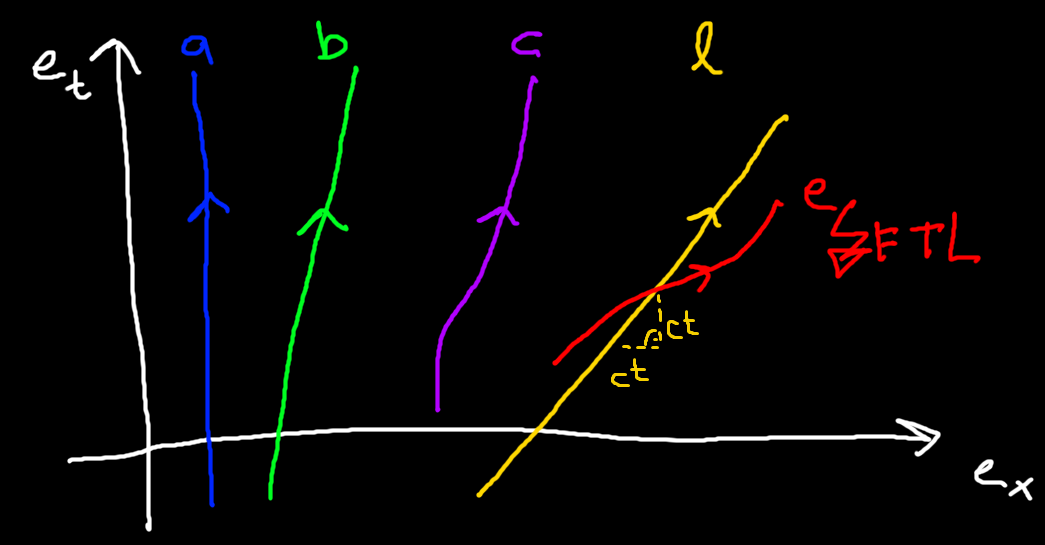Figure 5 - Paths in spacetime. Blue (a): Object at rest. Green (b): Object with constant velocity. Purple (c): Accelerated object. Yellow (l): Light. Red (e): Path faster than light.
##### Object at rest (a)
An object at rest does not move in space over time. Its path points purely in the time direction. The path can of course still be arbitrarily offset on the space axes.
The path velocity always points in the${e}_{t}$direction, so objects at rest will always have path velocity proportional to${e}_{t}$. Paths parameterized by proper time$\tau$will have path velocity$c{e}_{t}$because by definition, the path velocity for paths parameterized by proper time squares to${c}^{2}$. This will become very important later as objects at rest play an important role in Special Relativity.
##### Object with constant velocity (b)
Objects with constant velocity can move in space. Their path will be a rotated straight line. The more rotated the line is towards the space dimension, the faster the object goes.
The path velocity for an object moving along the x-direction will be some mix of${e}_{x}$and${e}_{t}$, although there are some restrictions to this.
##### Object with acceleration (c)
An object with acceleration could trace out a curved path like$c$in the diagram. Objects with non-zero acceleration won't be covered for now.
##### Light (l)
Light always moves at the speed of light. This is the second postulate of Special Relativity. Its path can be parameterized by$l\left(\lambda \right)=c\lambda {e}_{t}+c\lambda {e}_{x}$(the factor of$c$for the time dimension, as mentioned earlier, will be fully justified soon). This will trace out a 45° angle in our diagrams.
##### Faster than light (e)
Because nothing can move faster than light, this means all of our paths need to be steeper than 45°. Otherwise the object would be going faster than light.

#### Spacetime distance

Something we have not looked at yet is what a good notion of distance in spacetime is. Squaring vectors gives us vector lengths. When we do this, we make use of the squares of basis vectors. What should our spacetime basis vectors square to? To figure this out will perform a thought experiment involving light clocks and trains.
##### Light clocks and trains
First of all there are great videos demonstrating what we're about to investigate. You might want to watch them first or watch them if you get confused about the writing, the videos do a much better job. For example this one (although they put the device on the train instead of outside of it). We won't be using mirrors here as we can get the same result without two trips which also simplifies the math a bit.
Video 1 - Left: Apparatus as seen from Alice who is at rest with it. Right: Apparatus as seen from Bob who is moving relative to it.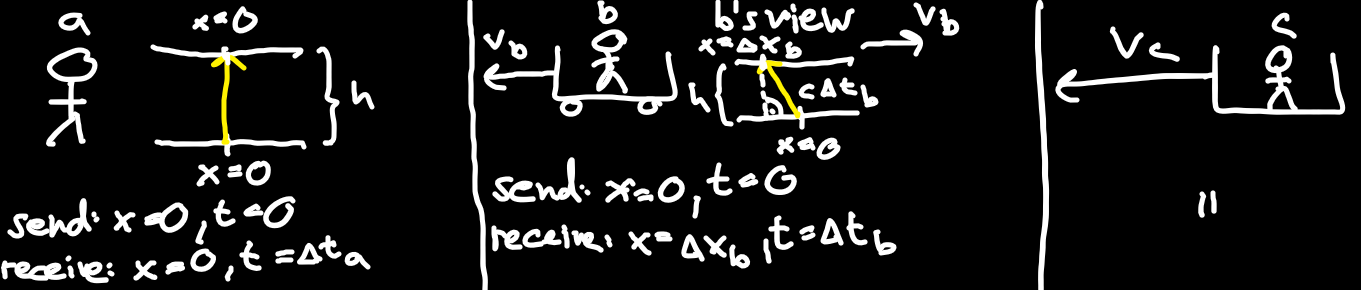Figure 6 - Left: Alice 'a' has a device that sends light from bottom and receives it at the top. Middle: Bob is on a moving train and looks at the device. Right: Charlie is on another moving train and looks at the device.
##### Setup and Alice
Consider Alice standing still on the ground with an apparatus as pictured in figure 6. The height of the apparatus is$h$. Light is sent from the bottom with horizontal coordinate$0$to the top in a straight line. In this case, the light is received at the top at the same horizontal coordinate it was sent from, ie.$0$. The time it took for the light to be sent and received is called$\mathrm{\Delta }{t}_{a}$.
Given the elapsed time we know that the distance the light moved must be equal to$c\mathrm{\Delta }{t}_{a}$where$c$is the speed of light. We also already knew that the height of the apparatus is$h$so we have
$\begin{array}{}\text{(5)}& h=c\mathrm{\Delta }{t}_{a}\end{array}$
##### Point of views and Bob's view
Introduce Bob on a train. From Alice's point of view the train is moving with constant velocity${v}_{b}$to the left. What exactly does "point of view" mean here? From our own point of view, we are always standing still and not moving in space. For example for Bob, for himself it looks like he is standing still on the train and Alice along with her apparatus are moving with velocity${v}_{b}$to the right.
How does the light in the aparatus look like from Bob's view? On sending, the light starts at horizontal coordinate$0$and is moving upwards in the aparatus. The second postulate of Special Relativity was that the speed of light is constant, so adding the speed of the train to the speed of light would not make sense. What happens in reality is that the aparatus keeps on moving so it slides away from the light, while the light just keeps moving straight up from Bob's point of view. When the light is received it is not received at the same horizontal coordinate anymore. If we look at the path the light traced out it is a diagonal. We denote the horizontal coordinate the light was received at${x}_{b}$and the time it took${t}_{b}$.
Let's look at the picture. Alice saw the light start and end at the coordinate$0$, yet Bob saw it start at$0$and end at${x}_{b}$. How is this possible? It seems unintuitive to everyday life but this is what actually happens. Using Pythagoras' theorem we can see that there is a relation between the total distance the light covered, the height of the apparatus and the horizontal distance
$\begin{array}{}\text{(6)}& {h}^{2}+\mathrm{\Delta }{x}_{b}^{2}=\left(c\mathrm{\Delta }{t}_{b}{\right)}^{2}\end{array}$
##### Invariant distance in Spacetime
A third person Charlie is also on a train, but moving at a different velocity${v}_{c}$. We will get an identical equation to Bob's
$\begin{array}{}\text{(7)}& {h}^{2}+\mathrm{\Delta }{x}_{c}^{2}=\left(c\mathrm{\Delta }{t}_{c}{\right)}^{2}\end{array}$
Solving Alice's, Bob's and Charlie's equations$\text{(5)}$,$\text{(6)}$,$\text{(7)}$for${h}^{2}$(which requires squaring Alice's equation) we get
$\begin{array}{rlrl}{h}^{2}& =& \left(c\mathrm{\Delta }{t}_{a}{\right)}^{2}& \\ {h}^{2}& =& \left(c\mathrm{\Delta }{t}_{b}{\right)}^{2}& -\left(\mathrm{\Delta }{x}_{b}{\right)}^{2}\\ {h}^{2}& =& \left(c\mathrm{\Delta }{t}_{c}{\right)}^{2}& -\left(\mathrm{\Delta }{x}_{c}{\right)}^{2}\end{array}$
All three right-hand sides must be equal. Does this look familiar? Think back to passive transformations. The coefficients of a vector expressed in a different coordinate basis might change, but the vector itself and its length does not change under passive transformations. This is exactly what happened here! There is a small but important difference in that they have a minus sign instead of a plus sign in front of the spatial offsets, so it is not just the ordinary euclidean distance we are dealing with here.
Note: Alice's part only appears to be missing because her spatial offset is zero (the light started and ended at the same horizontal coordinate).
In summary, all that happened was that the observers Alice, Bob and Charlie were using different coordinate systems, so the values they measured as expressed in their own coordinate systems did not match up, even though the thing they were measuring was fundamentally the same.
What we have discovered is the distance of spacetime that we can use to measure distance between spacetime events. With all three space dimensions it is
$\begin{array}{}\text{(8)}& {d}^{2}={c}^{2}\mathrm{\Delta }{t}^{2}-\mathrm{\Delta }{x}^{2}-\mathrm{\Delta }{y}^{2}-\mathrm{\Delta }{z}^{2}\end{array}$
If this quantity is preserved, then this also implies that the usual euclidean distance in spacetime is not preserved.
Using this result we can now see what changes we need to make to our 4D algebra to arrive at the correct Spacetime Algebra.

#### Spacetime Algebra

The only change we need to make is to the squares of our basis vectors. Having them all square to$+1$will give us the euclidean distance where all signs in the distance are positive. However we want the spatial signs to be negative, so naturally we choose the spatial basis vectors to square to -1.
${e}_{t}^{2}=1,{e}_{x}^{2}={e}_{y}^{2}={e}_{z}^{2}=-1$
This is usually refered to as the Spacetime Algebra. It has wide applications in physics and can be used to describe for example classical electromagnetics, most parts of the standard model of particle physics and, of course, relativity.
We can now verify that squaring a difference vector gives us the correct distance$\text{(8)}$:
$\begin{array}{rl}& ||c\mathrm{\Delta }t{e}_{t}+\mathrm{\Delta }x{e}_{x}+\mathrm{\Delta }y{e}_{y}+\mathrm{\Delta }z{e}_{z}|{|}^{2}=\\ =& \left(c\mathrm{\Delta }t{e}_{t}+\mathrm{\Delta }x{e}_{x}+\mathrm{\Delta }y{e}_{y}+\mathrm{\Delta }z{e}_{z}\right)\cdot \left(c\mathrm{\Delta }t{e}_{t}+\mathrm{\Delta }x{e}_{x}+\mathrm{\Delta }y{e}_{y}+\mathrm{\Delta }z{e}_{z}\right)=\\ =& {c}^{2}\mathrm{\Delta }{t}^{2}-\mathrm{\Delta }{x}^{2}-\mathrm{\Delta }{y}^{2}-\mathrm{\Delta }{z}^{2}\end{array}$
Now we also have a justification for the factor of$c$in front of the time component. Furthermore the algebra has the following basis blades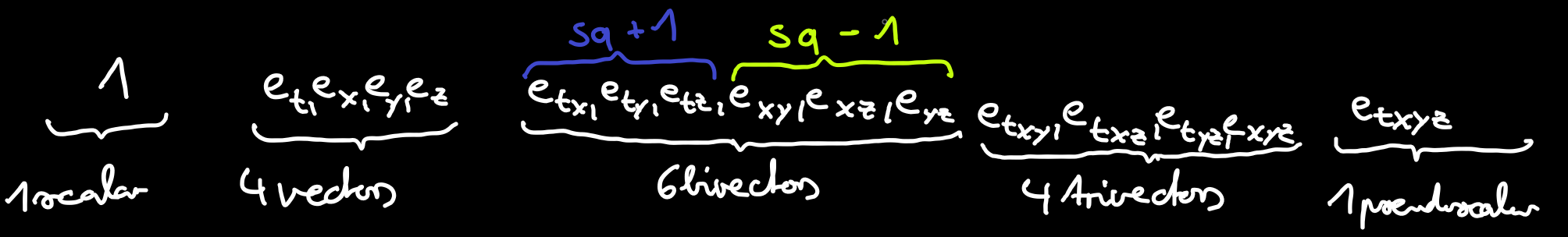Figure 7 - Basis blades of the Spacetime Algebra
In Geometric Algebra we are usually very interested in the bivectors as we can use them for building rotors that do interesting transformations which also easily compose. For example in ordinary Geometric Algebra the bivectors square to$-1$and the resulting rotors perform ordinary rotation.
Next we will take a look at a fundamental problem that relativity solves: the addition of velocities at speeds close to the speed of light. For this we will need to take a look at our bivectors squaring to$+1$and their rotors.

#### Conclusion

We started the section by looking at how we can express the paths objects take. We ended up with paths in spacetime parameterized by a parameter$\lambda$. Differentiating the path yields the path velocity tangent to the path. The path parameter is called$\tau$when the path velocity squares to${c}^{2}$.
We then saw that the points in spacetime are events containing both a space and time coordinate, and that we had to multiply our time component by the speed of light for the units to make sense.
After this we turned our attention to paths again and looked at different kinds of paths of objects in spacetime. Paths of objects at rest are straight lines in the${e}_{t}$direction. Paths with constant velocity are straight lines in both time and space directions. Light paths are at 45° angles in our diagrams and nothing can have an angle less steep than this.
Finally we performed a thought experiment involving a light clock and different observers going at different velocities relative to it to uncover a distance metric for our spacetime. This led to the introduction of the Spacetime Algebra with${e}_{t}$squaring to$1$and${e}_{x},{e}_{y},{e}_{z}$squaring to$-1$.

#### Formulas

• Path in spacetime:$s\left(\lambda \right)$
• Path velocity:$\frac{\partial }{\partial \lambda }s\left(\lambda \right)$
• Path velocity of object at rest:$\frac{\partial }{\partial \lambda }s\left(\lambda \right)\propto {e}_{t}$
• Path parameterized by proper time:$s\left(\tau \right),\stackrel{˙}{s}\left(\tau \right)=\frac{\partial }{\partial \tau }s\left(\tau \right),\stackrel{˙}{s}\left(\tau {\right)}^{2}={c}^{2}$
• Spacetime distance / invariant interval:${d}^{2}={c}^{2}\mathrm{\Delta }{t}^{2}-\mathrm{\Delta }{x}^{2}-\mathrm{\Delta }{y}^{2}-\mathrm{\Delta }{z}^{2}$
• Spacetime Algebra:${e}_{t}^{2}=1,{e}_{x}^{2}={e}_{y}^{2}={e}_{z}^{2}=-1$

#### Up next

Next we will look at a problem that arises when adding velocities close to the speed of light with ordinary addition, and we will see how the bivectors squaring to$+1$can be used to solve the problem.